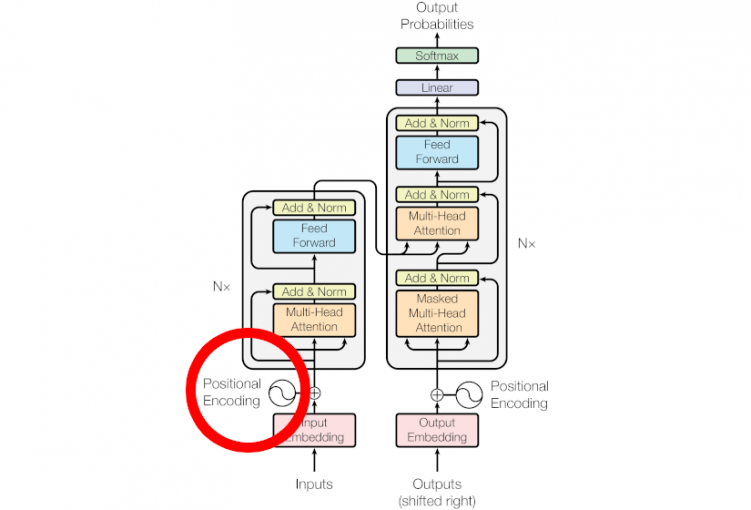# Linear Relationships in the Transformer’s Positional Encoding

· Timo Denk

In June 2017, Vaswani et al. published the paper “Attention Is All You Need” describing the “Transformer” architecture, which is a purely attention based sequence to sequence model. It can be applied to many tasks, such as language translation and text summarization. Since its publication, the paper has been cited more than one thousand times and several excellent blog posts were written on the topic; I recommend this one.

Vaswani et al. use positional encoding, to inject information about a token’s position within a sentence into the model. The exact definition is written down in section 3.5 of the paper (it is only a tiny aspect of the Transformer, as the red circle in the cover picture of this post indicates). After the definition, the authors state:

“We chose this function because we hypothesized it would allow the model to easily learn to attend by relative positions, since for any fixed offset $k$, $PE_{pos+k}$ can be represented as a linear function of $PE_{pos}$.”

But why is that? In this post I prove this linear relationship between relative positions in the Transformer’s positional encoding.

## Problem Statement

Let $\boldsymbol{E}\in\mathbb{R}^{n\times d_\text{model}}$ be a matrix that contains $d_\text{model}$-dimensional column vectors $\boldsymbol{E}_{t,:}$ which encode the position $t$ in an input sequence of length $n$. The function $e:\left\{1,\dots,n\right\}\rightarrow\mathbb{R}^{d_\text{model}}$ induces this matrix and is defined as
$$e(t) = \boldsymbol{E}_{t,:} := \begin{bmatrix} \sin\left(\frac{t}{f_1}\right)\\ \cos\left(\frac{t}{f_1}\right)\\ \sin\left(\frac{t}{f_2}\right)\\ \cos\left(\frac{t}{f_2}\right)\\ \vdots\\ \sin\left(\frac{t}{f_{\frac{d_\text{model}}{2}}}\right)\\ \cos\left(\frac{t}{f_{\frac{d_\text{model}}{2}}}\right) \end{bmatrix},\tag{1}$$ where the frequencies are
$$f_m = \frac{1}{\lambda_m} := 10000^{\frac{2m}{d_\text{model}}}.\tag{2}$$

The paper states that a linear transformation $\boldsymbol{T}^{(k)}\in\mathbb{R}^{d_\text{model}\times d_\text{model}}$ exists, for which
$$\boldsymbol{T}^{(k)}\boldsymbol{E}_{t,:}=\boldsymbol{E}_{t+k,:}\tag{3}$$holds for any positional offset $k\in\left\{1,\dots,n\right\}$ at any valid position $t\in\left\{1,\dots,n-k\right\}$ in the sequence.

## Derivation

That is true, because a definition for $\boldsymbol{T}^{(k)}$ can be found with no dependence on $t$:
$$\boldsymbol{T}^{(k)} = \begin{bmatrix} \boldsymbol{\Phi}^{(k)}_1 & \boldsymbol{0} & \cdots & \boldsymbol{0} \\ \boldsymbol{0} & \boldsymbol{\Phi}^{(k)}_2 & \cdots & \boldsymbol{0} \\ \boldsymbol{0} & \boldsymbol{0} & \ddots & \boldsymbol{0} \\ \boldsymbol{0} & \boldsymbol{0} & \cdots & \boldsymbol{\Phi}^{(k)}_{\frac{d_\text{model}}{2}} \end{bmatrix},\tag{4}$$ where $\boldsymbol{0}$ denotes $2\times2$ all-zero matrices and the #$\frac{d_\text{model}}{2}$ transposed rotation matrices $\boldsymbol{\Phi}^{(k)}$ positioned on the main diagonal are defined by
$$\boldsymbol{\Phi}^{(k)}_m = \begin{bmatrix} \cos(r_mk) & -\sin(r_mk) \\ \sin(r_mk) & \cos(r_mk) \end{bmatrix}^\intercal,\tag{5}$$with wave length $r_m$ (not to be confused with the encoding wave length $\lambda_m$).

Now we can reformulate Eq. 3, which we want to prove, as
$$\underbrace{\begin{bmatrix} \cos(r_mk) & \sin(r_mk) \\ -\sin(r_mk) & \cos(r_mk) \end{bmatrix}}_{\displaystyle\boldsymbol{\Phi}^{(k)}_m} \begin{bmatrix} \sin\left(\lambda_mt\right)\\ \cos\left(\lambda_mt\right) \end{bmatrix} = \begin{bmatrix} \sin\left(\lambda_m\left(t+k\right)\right)\\ \cos\left(\lambda_m\left(t+k\right)\right) \end{bmatrix}.\tag{6}$$Expanded, that is
\begin{align} \sin(\lambda k+\lambda t) &= \sin(rk)\cos(\lambda t)+\cos(rk)\sin(\lambda t)\tag{6a}\\ \cos(\lambda k+\lambda t) &= \cos(rk)\cos(\lambda t)-\sin(rk)\sin(\lambda t),\tag{6b} \end{align}and we seek to determine $r$ in dependence of $\lambda$ and $k$, while eliminating $t$.

The addition theorems\begin{align} \sin \left( {\alpha + \beta } \right) &= \sin \alpha \cos \beta + \cos \alpha \sin \beta\tag{7a}\\ \cos \left( {\alpha + \beta } \right) &= \cos \alpha \cos \beta – \sin \alpha \sin \beta\tag{7b} \end{align}help solving the problem at hand. When applying the addition theorems to the expanded form (Eq. 6a, 6b), it follows\begin{align} \alpha&=\lambda k=rk\tag{8a}\\ \beta&=\lambda t=\lambda t,\tag{8b} \end{align}and consequently $r=\lambda$. Applying that finding and the definition of $\lambda_m$ (Eq. 2) to Eq. 5, we get
$$\boldsymbol{\Phi}^{(k)}_m = \begin{bmatrix} \cos\left(\lambda_mk\right) & \sin\left(\lambda_mk\right) \\ -\sin\left(\lambda_mk\right) & \cos\left(\lambda_mk\right) \end{bmatrix},\tag{9}$$ where $\displaystyle\lambda_m=10000^{\frac{-2m}{d_\text{model}}}$. With that, $\boldsymbol{T}^{(k)}$ fully specified and depends solely on $m$, $d_\text{model}$, and $k$. The position within the sequence, $t$, is not a parameter, q.e.d.

Special thanks go to my colleagues Stefan Baur and Lennart Van der Goten, for their friendly support and readiness to share their knowledge.